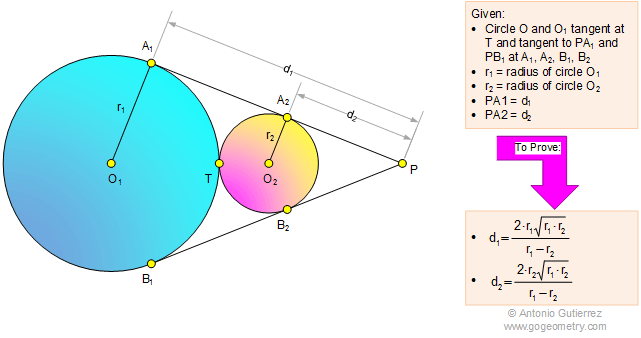Problem 1095: Externally Tangent Circles, Common Tangent, Radius, Geometric Mean, Metric Relations. Level: High School, SAT Prep, College Geometry

< PREVIOUS PROBLEM  |  NEXT PROBLEM >

 The figure shows the circles O1 (radius r1) and O2 (radius r2) tangent at T and tangent to PA1 and PB1 at A1, A2, B1, and B2, respectively. If PA1 = d1 and PA2 = d2, prove that (1); (2).Home | Search | Geometry | Problems | All Problems | Open Problems | Visual Index | 1091-1100 | Circle | Tangent Line | Tangent Circles | Problem 454 | Geometric Mean | Email | Post a comment or solution | By Antonio GutierrezLast updated: Mar 10, 2015